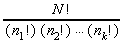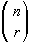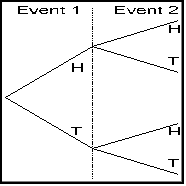Counting Techniques

Fundamental Theorems

Every branch of mathematics has its fundamental theorem or theorems.

Fundamental Theorem of Arithmetic

Every integer greater than one is either prime or can be expressed as an unique product of prime numbers

Fundamental Theorem of Algebra

Every polynomial function on one variable of degree n > 0 has at least one real or complex zero.

Fundamental Theorem of Linear Programming

If there is a solution to a linear programming problem, then it will occur at a corner point or on a boundary between two or more corner points

Fundamental Counting Principle

In a sequence of events, the total possible number of ways all events can performed is the product of the possible number of ways each individual event can be performed.

Factorials

If n is a positive integer, then

n! = n (n-1) (n-2) ... (3)(2)(1)
n! = n (n-1)!

A special case is 0!

0! = 1

Permutations

A permutation is an arrangement of objects without repetition and where order is important.

Another definition of permutation is the number of arrangements that can be formed.

Permutations using all the objects

A permutation of n objects, arranged into one group of size n, without repetition, and order being important is:

nPn = P(n,n) = n!

Example: Find all permutations of the letters "ABC"

ABC  ACB  BAC  BCA  CAB  CBA

Permutations of some of the objects

A permutation of n objects, arranged in groups of size r, without repetition, and order being important is:

nPr = P(n,r) = n! / (n-r)!

The calculator can be used to find the number of such permutations. On the TI-82 or TI-83, the permutation key is found under the Math, Probability menu.

Example: Find all two-letter permutations of the letters "ABC"

AB  AC  BA  BC  CA  CB
Shortcut formula for finding a permutation

Assuming that you start a n and count down to 1 in your factorials ...

P(n,r) = first r factors of n factorial

Distinguishable Permutations

Sometimes letters are repeated and all of the permutations aren't distinguishable from each other.

Example: Find all permutations of the letters "BOB"

BOb  BbO  OBb  ObB  bBO  bOB

If you just write "B" as "B", however ...

BOB  BBO  OBB  OBB  BBO  BBO

There are really only three distinguishable permutations here.

BOB  BBO  OBB

If a word has N letters, k of which are unique, and you let n (n1, n2, n3, ..., nk) be the frequency of each of the k letters, then the total number of distinguishable permutations is given by:Consider the word "STATISTICS":

Here are the frequency of each letter: S=3, T=3, A=1, I=2, C=1, there are 10 letters total

10!        10*9*8*7*6*5*4*3*2*1
Permutations = -------------- = -------------------- = 50400
3! 3! 1! 2! 1!    6 * 6 * 1 * 2 * 1

You can find distinguishable permutations using the TI-82.

Combinations

A combination is an arrangement of objects without repetition and where order is not important.

Note: The difference between a permutation and a combination is not whether there is repetition or not - there must not be repetition with either, and if there is repetition, you can not use the formulas for permutations or combinations. The only difference in the definition of a permutation and a combination is whether order is important.

A combination of n objects, arranged in groups of size r, without repetition, and order being important is:

nCr = C(n,r) = n! / ( (n-r)! * r! )

Another way to write a combination of n things, r at a time is using the binomial notation:Example: Find all two-letter combinations of the letters "ABC"

AB = BA   AC = CA   BC = CB

There are only three two-letter combinations.

Shortcut formula for finding a combination

Assuming that you start a n and count down to 1 in your factorials ...

C(n,r) = first r factors of n factorial divided by the last r factors of n factorial

Pascal's Triangle

Combinations are used in the binomial expansion theorem from algebra to give the coefficients of the expansion (a+b)^n. They also form a pattern known as Pascal's Triangle.

1
1   1
1   2   1
1   3   3   1
1   4   6   4   1
1   5  10   10  5   1
1   6  15  20  15   6   1
1   7  21  35  35  21   7   1

Each element in the table is the sum of the two elements directly above it. Each element is also a combination. The n value is the number of the row (start counting at zero) and the r value is the element in the row (start counting at zero). That would make the 20 in the next to last row C(6,3) -- it's in the row #6 (7th row) and position #3 (4th element).

Symmetry

Pascal's Triangle illustrates the symmetric nature of a combination. C(n,r) = C(n,n-r)

Example: C(10,4) = C(10,6) or C(100,99) = C(100,1)

Shortcut formula for finding a combination

Since combinations are symmetric, if n-r is smaller than r, then switch the combination to its alternative form and then use the shortcut given above.

C(n,r) = first r factors of n factorial divided by the last r factors of n factorial

TI-82

You can use the TI-82 graphing calculator to find factorials, permutations, and combinations.

Tree DiagramsTree diagrams are a graphical way of listing all the possible outcomes. The outcomes are listed in an orderly fashion, so listing all of the possible outcomes is easier than just trying to make sure that you have them all listed. It is called a tree diagram because of the way it looks.

The first event appears on the left, and then each sequential event is represented as branches off of the first event.

The tree diagram to the right would show the possible ways of flipping two coins. The final outcomes are obtained by following each branch to its conclusion: They are from top to bottom:

HH  HT  TH  TT